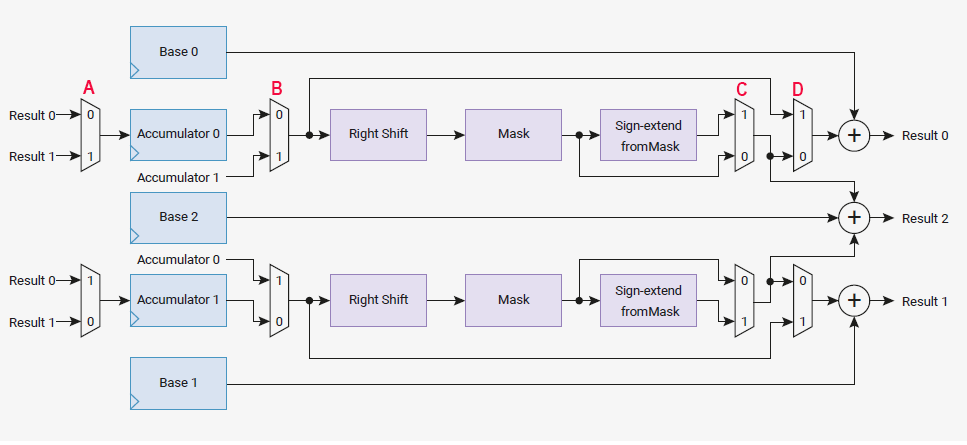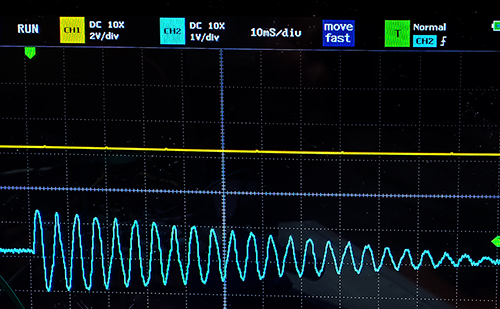Cornell University ECE4760
Interpolator

Pi Pico RP2040

The FM sine generation on the interpolator subsystem
The rp2040 has two fairly specialized interpolator hardware modules (interp) for each M0 core. The advantage of using the interp is that each unit can perform an add, shift, bit-mask, and a second add on each cpu clock cycle. Data paths are set up by configuring registers. The setup requires bending your brain to figure out the intent of the interp design. My first attempt was to convert a Direct Digital Synthesis algorithm (DDS) to do as much as possible on the interp. Two of the DDS generators were used to generate a FM synthesis.
The basic waveform equation for FM is:
output wave = sin(Fout*t + fm_depth*(sin(Fmod*t)))
which requires two DDS sine generators.

The basic idea is that the DDS phase accumulator can be mapped to interp acumulator0, with the increment in base0, and setting the D mux below to 1, and the A and B muxes to 0. The DDS mapping of the phase accumulaor to sine table index is done by the right-shift and mask, but without sign extend by setting the C mux below to 0. Base2 contains a pointer to the beginning of the sine table, which is added to the index and appears at result2. (Refering to the diagram below). In this example, interp0 lane1 (accum1) is not used. The modulation frequency is generated by interpolator0, and the final wave form is generated by interpolator1, using input from the sine wave generated by interp0.Image is slightly modified from the RP2040 hardware manual.

The configuration for FM using DDS is:

Interp0 data:
-- accum0 holds the modulation frequency DDS phase.
-- base0 holds the modulation frequency DDS increment.
-- base2 is a pointer to mod_sine_table base address.
Interp0 (FM modulation frequency) is setup to:
-- add accum0 + base0 and store result in accum0 (result0 to accum0) (add raw -- no shift)
This data path implements the Fmod DDS phase increment.
-- right-shift accum0 23 bits and mask to bits 8:1 (zero low bit for short pointer)
This implements the DDS determination of the sine table index
This implements the DDS sine table address a the index determined above
-- The C progam will read result2 as sine table address and copy (shifted) table value
plus the Fout increment to interp1_base0.
The shift operation imlements the fm_depth multiply in the above equation.

Interp1 data:
-- accum0 holds the main frequency DDS phase
-- base0 holds the main frequency (Fout) DDS increment (sum of Fout increment and Fmod inc)
-- base2 is a pointer to sine_table base address
Interp1 (main oscillator) setup to:
-- add accum0 + base0 amd store in accum0 (result0 to accum0) (add raw -- no shift)
-- right-shift accum0 23 bits and mask to bits 8:1 (zero low bit for short pointer)/
-- The C progam will read result2 as table position output and copy table value to PWM

The actual setup code for interpolators is short, but obscure.
Refer often to the register diagram above and to the C_SDK sections 4.1.11 and 4.1.12.

The thread which sequences the interpolators runs at Fs rate. The obscure aspects of this code are that reading any pop register on the interpolators clocks the next result in. For instance, reading interp0->pop reads out the contents of the result2 register and clocks interp0. Also, the integer read from the register needs to be cast to a pointer-to-short, then accessed, then cast to int.

Adding a simple amplitude envelope using an interpolator
Most sounds are recognized both by their spectral content and by their time course. The FM generator described above was modified to use another interp lane to produce a simple decaying amplitude envelope. The envelope is set to some maximum value by the C code by initializing an accumulaor. A constant in a base register is subtracted on each synthesis sample until the envelope amplitude is zero. The basic waveform equation for this process is:
output wave = (amp_envelope(t)) * sin(Fout*t + fm_depth*(sin(Fmod*t)))

The setup code configures three lanes.
Interpolator0-lane1 (FM modulation frequency) setup to:
-- add accum1 + base1 amd store in accum0 (result1 to accum1) (add raw -- no shift)
-- right-shift accum1 23 bits and mask to bits 8:1 (zero low bit for short pointer)

Interpolator0-lane0 (AM modulation amplitude) setup to:
-- add accum0 + base0 amd store in accum0 (result0 to accum0) (add raw -- no shift)
but note that base0 contains a negative number.

Interpolator1-lane1 (main oscillator) setup to:
-- add accum1 + base1 amd store in accum0 (result1 to accum1) (add raw -- no shift)
-- right-shift accum1 23 bits and mask to bits 8:1 (zero low bit for short pointer)

For a main frequency of 200 Hz, modulation frequency of 330 Hz,
fm_depth of 16 and decay rate of 100 we get the following waveform.
You can see the linear decay and the odd distortion due to the FM modulation.It would be possible to add one more amplitude modulation (perhaps rise time)
using the interpolators, but the C overhead makes it less desirable. A more general system
is described below with the DDS units in the interpolators and amplitude envelope in C.

FM synthesizer with attack, sustain, decay envelope controls
The interpolators are used for the FM DDS, but the amplitude is set similarly to the scheme used on the PIC32. While the interpolators are using integer counter arithmetic, most of the envelope calculations will be done in s15x16 fixed point. Also, the relatively coarse fm_depth setting of the pervious examples, using shifts, will be replaced with actual fixed-point multiplies.

Copyright Cornell University June 18, 2022